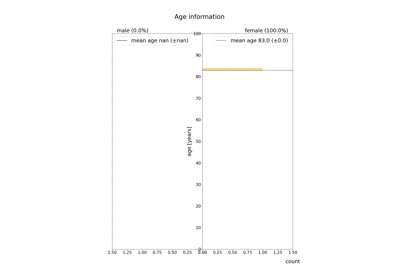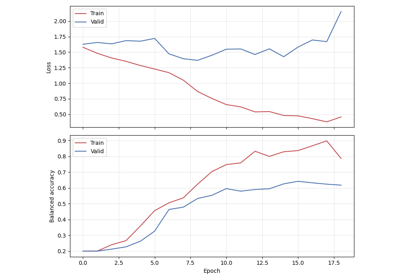# braindecode.preprocessing.scale#

braindecode.preprocessing.scale(data, factor)[source]#

DEPRECATED: will be removed in 0.8.0. Use numpy.multiply inside a lambda function instead.

Scale continuous or windowed data in-place

Parameters
• data (np.ndarray (n_channels x n_times) or (n_windows x n_channels x) –

• n_times) – continuous or windowed signal

• factor (float) – multiplication factor

Returns

• scaled (np.ndarray (n_channels x n_times) or (n_windows x n_channels x)

• n_times) – normalized continuous or windowed data

• ..note – If this function is supposed to preprocess continuous data, it should be given to raw.apply_function().

## Examples using `braindecode.preprocessing.scale`#Process a big data EEG resource (TUH EEG Corpus)

Process a big data EEG resource (TUH EEG Corpus)Sleep staging on the Sleep Physionet dataset using Chambon2018 network

Sleep staging on the Sleep Physionet dataset using Chambon2018 network